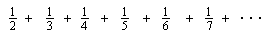## Answers for Chapter 5 Sample Problems

The harmonic serieslooks innocent enough. My students used their calculators and even wrote computer programs to look at the partial sums. One day some youngsters must have gotten the sum of the first 10,000 terms, and it hadn't reached 10! They were ready to say the infinite sum went to 10 as the limit. I have since received four responses about this, from these very helpful people around the world - Karl Cooper, Gabriel Lombardi and Renwei Mei. Using 'double precision' (#) finally, in the QBasic program: s#=0; for n= 2 to 1000000; s#=s#+1#/n; next n; print s#; end, we get 13.39272672286481 for the sum of the first 999999 terms of the Harmonic Series.

Ms. Cohei wrote in an email from Japan:

"Incidentally, I wrote a program for the Harmonic Series in C language. Just for reference your information, here it is:

#include <stdio.h>

main()
{
double n,i,sum;
sum=0;
printf("n=");scanf("%lf",&n);
for(i=2;i<=n+1;i++)
sum=sum+1/i;
printf("sum(%.0lf)=%.15lf\n",n,sum);
return(0);
}

The results:

C:\Borland\bcc55\Bin>harmonic
n=100000000
sum(100000000)=17.997896423849664

C:\Borland\bcc55\Bin>harmonic
n=1000000000
sum(1000000000)=20.300481503508941

I was very excited with this program because it could calculate the sum total of one billion terms of the Harmonic Series !

Thank you very much. Cohei"

It's wonderful to hear from people who are really reading my books and using them with their children. It's nice to know I'm not perfect, and I'm always learning new things. This series tends to infinity, diverges, but very slowly.

In about 1350 Oresme proved this series to be divergent. The proof is based on the idea that pieces of the harmonic series are > 1/2 and the series 1/2 + 1/2 + 1/2 +... diverges, the harmonic series also diverges. See my worksheet book for the proof.

Back to Ch. 5 problems